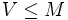# Homocyclic normal implies finite-pi-potentially fully invariant in finite

This article gives the statement and possibly, proof, of an implication relation between two subgroup properties, when the big group is a finite group. That is, it states that in a Finite group (?), every subgroup satisfying the first subgroup property (i.e., Homocyclic normal subgroup (?)) must also satisfy the second subgroup property (i.e., Finite-pi-potentially fully invariant subgroup (?)). In other words, every homocyclic normal subgroup of finite group is a finite-pi-potentially fully invariant subgroup of finite group.
View all subgroup property implications in finite groups$|$ View all subgroup property non-implications in finite groups$|$ View all subgroup property implications$|$ View all subgroup property non-implications

## Statement

Let$G$ be a finite group and$H$ be a homocyclic normal subgroup of$G$. In other words,$H$ is a normal subgroup of$G$ and is also a homocyclic group: it is a direct product of pairwise isomorphic cyclic groups. Then, there exists a finite group$K$ containing$G$ such that all the prime factors of the order of$K$ also divide the order of$G$, and$H$ is a fully invariant subgroup of$K$.

## Proof

Given: A finite group$G$, a homocyclic normal subgroup$H$ of$G$.

To prove: There exists a finite group$K$ containing$G$ such that$H$ is a fully invariant subgroup of$K$.

Proof: Let$m$ be the exponent of$H$. The order of$H$ is thus$m^d$ for some$d$ ($d$ is the number of copies of the cyclic group). Let$n$ be the order of$G$. Let$h$ be the unique largest divisor of$n$ that is relatively prime to$m$. Let$k$ be chosen such that$n/h$ divides$m^{k-1}$.

Let$M$ be the homocyclic group of order$m^{dk}$, with exponent$m^k$. In other words,$M$ is the direct product of$d$ copies of the cyclic group of order$m^k$.$H$ sits inside$M$ as the set of elements whose order divides$m$. The induced action by conjugation of$G/H$ on$H$ extends to an action of$G/H$ on$M$. By fact (1), we get a group$K$ containing$G$ and$M$ with$G \cap M = H$,$GM = K$, and the action of$K/M \cong G/H$ on$M$ equal to the chosen extension of the action on$H$. Note that the order of$K$ is$m^{dk}(n/m)$ which has no prime factors other than those of$G$.

Let$V$ be the subgroup of$K$ generated by all elements of the form$x^{hm^{k-1}}$. Then,$V$ is a verbal subgroup, hence a fully invariant subgroup.$V \le M$, because the order of$K/M \cong G/H$ divides$n$ which divides$hm^{k-1}$ by assumption. Also,$H \le V$, because$H$ is the set$\{ x^{hm^{k-1}} \mid x \in K \}$. So,$H \le V \le M$.

Further,$H$ is a fully invariant subgroup of$V$, since it is the set of elements whose order divides$m$. Thus, by fact (2),$H$ is fully invariant in$K$.